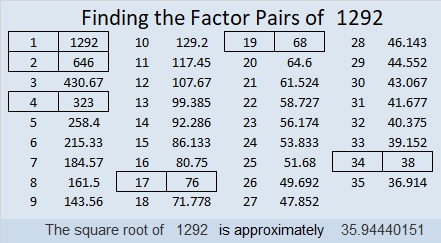# 1292 and Level 4

Finding the Factors in this puzzle will require you to use logic and you will get a bonus: your knowledge of the multiplication table will be a little better than it was before.Print the puzzles or type the solution in this excel file: 12 factors 1289-1299

Now I’ll give you some information about today’s puzzle number:

• 1292 is a composite number.
• Prime factorization: 1292 = 2 × 2 × 17 × 19, which can be written 1292 = 2² × 17 × 19
• The exponents in the prime factorization are 2, 1, and 1. Adding one to each and multiplying we get (2 + 1)(1 + 1)(1 + 1) = 3 × 2 × 2 = 12. Therefore 1292 has exactly 12 factors.
• Factors of 1292: 1, 2, 4, 17, 19, 34, 38, 68, 76, 323, 646, 1292
• Factor pairs: 1292 = 1 × 1292, 2 × 646, 4 × 323, 17 × 76, 19 × 68, or 34 × 38
• Taking the factor pair with the largest square number factor, we get √1292 = (√4)(√323) = 2√323 ≈ 36.94441292 is the hypotenuse of a Pythagorean triple:
608-1140-1292 which is (8-15-17) times 76